Next: Mean Place to Apparent Place
Up: EXPLANATION AND EXAMPLES
Previous: Different Sorts of Mean Place

## Mean Place Transformations

Figure 1 is based upon three varieties of mean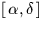all of which are of practical significance to observing astronomers in the present era:
• Old style (FK4) with known proper motion in the FK4 system, and with parallax and radial velocity either known or assumed zero.
• Old style (FK4) with zero proper motion in FK5, and with parallax and radial velocity assumed zero.
• New style (FK5) with proper motion, parallax and radial velocity either known or assumed zero.
The figure outlines the steps required to convert positions in any of these systems to a J2000for the current epoch, as might be required in a telescope-control program for example. Most of the steps can be carried out by calling a single SLALIB routines; there are other SLALIB routines which offer set-piece end-to-end transformation routines for common cases. Note, however, that SLALIB does not set out to provide the capability for arbitrary transformations of star-catalogue data between all possible systems of mean.Only in the (common) cases of FK4, equinox and epoch B1950, to FK5, equinox and epoch J2000, and vice versa are proper motion, parallax and radial velocity transformed along with the star position itself, the focus of SLALIB support.

As an example of using SLALIB to transform mean places, here is a program which implements the top-left path of Figure 1. An FK4of arbitrary equinox and epoch and with known proper motion and parallax is transformed into an FK5 J2000for the current epoch. As a test star we will use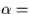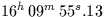,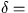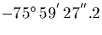, equinox 1900, epoch 1963.087,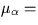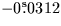/y,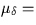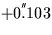/y, parallax =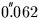, radial velocity = -34.22 km/s. The epoch of observation is 1994.35.

            IMPLICIT NONE
DOUBLE PRECISION AS2R,S2R
PARAMETER (AS2R=4.8481368110953599D-6,S2R=7.2722052166430399D-5)
INTEGER J,I
DOUBLE PRECISION R0,D0,EQ0,EP0,PR,PD,PX,RV,EP1,R1,D1,R2,D2,R3,D3,
:                 R4,D4,R5,D5,R6,D6,EP1D,EP1B,W(3),EB(3),PXR,V(3)
DOUBLE PRECISION sla_EPB,sla_EPJ2D

*  RA, Dec etc of example star
CALL sla_DTF2R(16,09,55.13D0,R0,J)
CALL sla_DAF2R(75,59,27.2D0,D0,J)
D0=-D0
EQ0=1900D0
EP0=1963.087D0
PR=-0.0312D0*S2R
PD=+0.103D0*AS2R
PX=0.062D0
RV=-34.22D0
EP1=1994.35D0

*  Epoch of observation as MJD and Besselian epoch
EP1D=sla_EPJ2D(EP1)
EP1B=sla_EPB(EP1D)

*  Space motion to the current epoch
CALL sla_PM(R0,D0,PR,PD,PX,RV,EP0,EP1B,R1,D1)

*  Remove E-terms of aberration for the original equinox
CALL sla_SUBET(R1,D1,EQ0,R2,D2)

*  Precess to B1950
R3=R2
D3=D2
CALL sla_PRECES('FK4',EQ0,1950D0,R3,D3)

*  Add E-terms for the standard equinox B1950

*  Transform to J2000, no proper motion
CALL sla_FK45Z(R4,D4,EP1B,R5,D5)

*  Parallax
CALL sla_EVP(sla_EPJ2D(EP1),2000D0,W,EB,W,W)
PXR=PX*AS2R
CALL sla_DCS2C(R5,D5,V)
DO I=1,3
V(I)=V(I)-PXR*EB(I)
END DO
CALL sla_DCC2S(V,R6,D6)
:


It is interesting to look at how thechanges during the course of the calculation:


16 09 55.130 -75 59 27.20 		 		 original equinox and epoch
16 09 54.155 -75 59 23.98 		 		 with space motion
16 09 54.229 -75 59 24.18 		 		 with old E-terms removed
16 16 28.213 -76 06 54.57 		 		 precessed to 1950.0
16 16 28.138 -76 06 54.37 		 		 with new E-terms
16 23 07.901 -76 13 58.87 		 		 J2000, current epoch
16 23 07.907 -76 13 58.92 		 		 including parallax


Other remarks about the above (unusually complicated) example:

• If the original equinox and epoch were B1950, as is quite likely, then it would be unnecessary to treat space motions and E-terms explicitly. Transformation to FK5 J2000 could be accomplished simply by calling sla_FK425, after which a call to sla_PM and the parallax code would complete the work.
• The rigorous treatment of the E-terms has only a small effect on the result. Such refinements are, nevertheless, worthwhile in order to facilitate comparisons and to increase the chances that star positions from different suppliers are compatible.
• The FK4 to FK5 transformations, sla_FK425 and sla_FK45Z, are not as is sometimes assumed simply 50 years of precession, though this indeed accounts for most of the change. The transformations also include adjustments to the equinox, a revised precession model, elimination of the E-terms, a change to the proper-motion time unit and so on. The reason there are two routines rather than just one is that the FK4 frame rotates relative to the background, whereas the FK5 frame is a much better approximation to an inertial frame, and zero proper motion in FK4 does not, therefore, mean zero proper motion in FK5. SLALIB also provides two routines, sla_FK524 and sla_FK54Z, to perform the inverse transformations.
• Some star catalogues (FK4 itself is one) were constructed using slightly different procedures for the polar regions compared with elsewhere. SLALIB ignores this inhomogeneity and always applies the standard transformations irrespective of location on the celestial sphere.Next: Mean Place to Apparent Place
Up: EXPLANATION AND EXAMPLES
Previous: Different Sorts of Mean Place

SLALIB --- Positional Astronomy Library